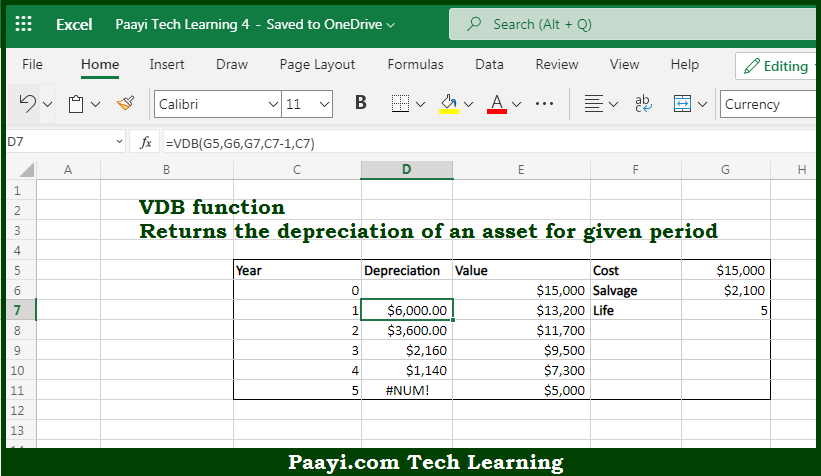# Learn How to Use Microsoft Excel VDB Function

Written by | 0 Comments | 350 Views

In this article, you will learn how to use the Microsoft Excel VDB function and its prime function in Microsoft Excel. You will also get to know the Microsoft Excel VDB function return value and syntax with the help of some examples.

Microsoft Excel VDB Function

The main purpose of the Microsoft Excel VDB function is to get the depreciation for the double-declining variable. That implies, with the help of the VDB function you can able to return the depreciation of an asset for the given period. In this function, the double-declining balance method or another method is used which is specified by changing the factor argument. It should be noted that the VDB function will switch to a straight-line calculation by default. VDB is the abbreviation for the variable declining balance. So, with the help of the VDB function, you can able to get depreciation for the double-declining variable.

Return Value of VDB Function

The return value will be the depreciation for the specified period.

Syntax of VDB Function

=VDB(cost, salvage, life, start, end, [factor], [no-switch])

Where the arguments:

• cost: This is the initial cost of the asset.
• salvage: This is the asset value at the end of the depreciation.
• life: This is the period over which an asset is depreciated.
• start: This is the start period.
• end: This is the end period.
• factor: This is the rate at which the balance declines, and In case omitted, defaults to 2 (optional).
• no-switch: It doesn't switch to the straight line, and the default is FALSE (optional).

How to Use Microsoft Excel VDB Function?So we know that Microsoft Excel VDB function you can able to get the depreciation for the double-declining variable. That implies, with the help of the VDB function you can able to return the depreciation of an asset for the given period. In this function, the double-declining balance method or another method is used which is specified by changing the factor argument. It should be noted that the VDB function will switch to the straight-line calculation by default. VDB is the abbreviation for the variable declining balance. So, with the help of the VDB function, you can able to get depreciation for the double-declining variable.